# Chapter 1: Applications of Matrices and Determinants - Online Test

Q1. If | adj(adj A) |=| A |9 , then the order of the square matrix A is
Explaination / Solution: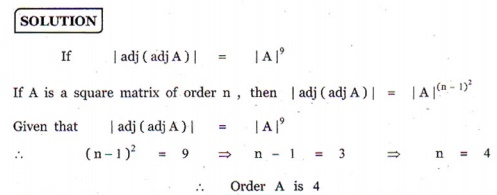Q2. If A is a 3× 3 non-singular matrix such that AAT = AT A and B = A1 AT , then BBT =
Explaination / Solution: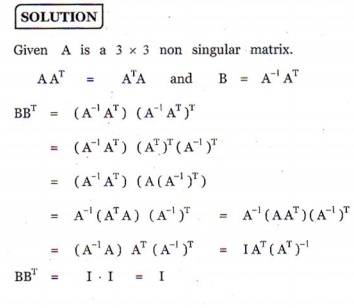Q3. If A =, B = adj A and C = 3A , then=
Explaination / Solution: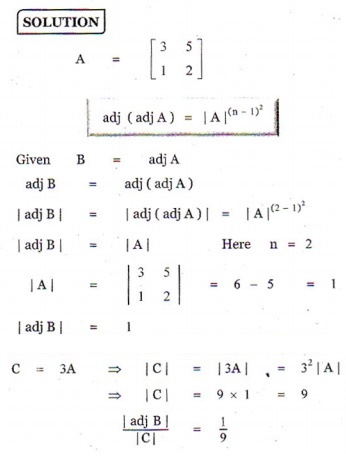Q4. if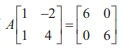, then A =
Explaination / Solution:Q5. if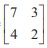, then 9I2 – A =
Explaination / Solution: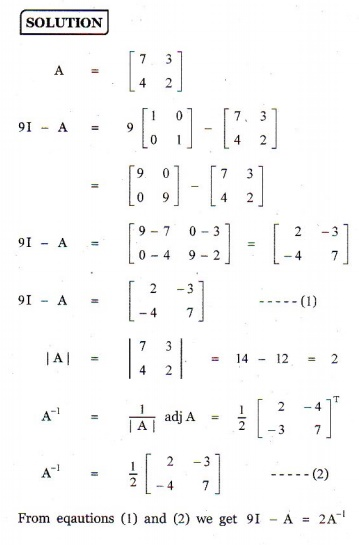Q6. if A =, B=then | adj ( AB) |=
Explaination / Solution: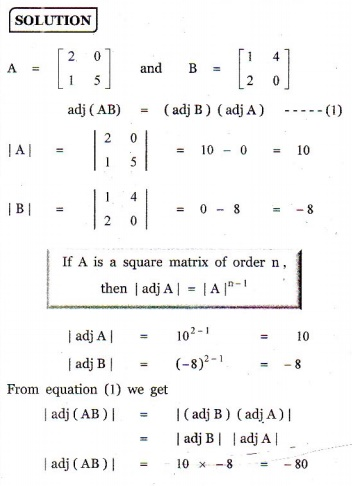Q7. If P=is the adjoint of 3× 3 matrix A and | A |= 4 , then x is
Explaination / Solution:Q8. If A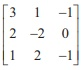, and A1then the value of a23 is
Explaination / Solution: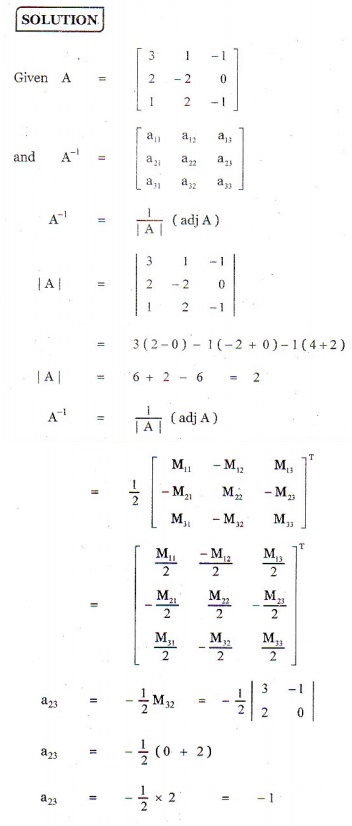Q9. If A, B and C are invertible matrices of some order, then which one of the following is not true?
Explaination / Solution: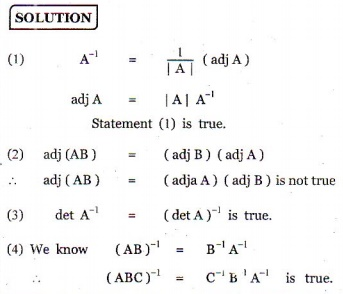Q10. If ( AB)1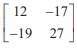, and A-1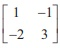, then B1 =
Explaination / Solution: